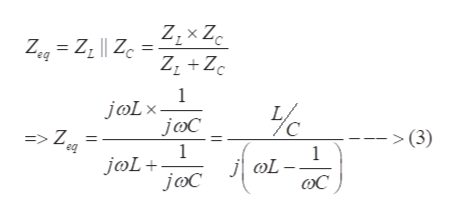# A resistor of 5 ohm resistance is connected in series with a parallel combination of an inductor of 2 H and a capacitor of 0.5 F. The angular frequency of the input ac is 2 rad/s. What will be total impedance of the combination?

Question
1 views

A resistor of 5 ohm resistance is connected in series with a parallel combination of an inductor of 2 H and a capacitor of 0.5 F. The angular frequency of the input ac is 2 rad/s.

What will be total impedance of the combination?

check_circle

star
star
star
star
star
1 Rating
Step 1

The impedance of an inductor of inductance L H can be expressed as

Step 2

The impedance of a capacitor of capacitance C F can be expressed as

Step 3

Since the inductor and the capacitor are in parallel to each other,...help_outlineImage TranscriptioncloseZeg Z Zc 1 joLx => Z. eq >(3) 1 joL joC 1 L fullscreen

### Want to see the full answer?

See Solution

#### Want to see this answer and more?

Solutions are written by subject experts who are available 24/7. Questions are typically answered within 1 hour.*

See Solution
*Response times may vary by subject and question.
Tagged in

### Electrical Engineering